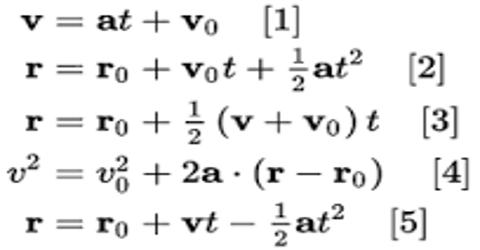# Fifth Equation of Dimensional Motion

Fifth equation

Let the initial velocity of an object moving with uniform acceleration ‘a’ be v0. We need to find the distance traveled by the object at a particular second. Let the distance traveled at t-th second be st. It is equal to the difference between the distances traveled by the object in time t second and (t – 1) second.

st = distance traveled in t sec — distance traveled in (t – 1) sec.

= (v0t + ½ at2) – {v0 (t-1) + ½ a (t-1)2} (from forth equation)

= v0t + ½ at2 – {v0t – v0 + ½ a (t2 – 2t + 1)}

= v0t + ½ at2 – {v0t – v0 + ½ a t2 – at + ½ a)}

= v0t + ½ at2 – v0t + v0 – ½ a t2 + at – ½ a

= v0 + at – ½ a = v0 + ½ a (2t – 1)

so, st = v0 + ½ a (2t – 1) … … … (1)

If the object start from rest, then v0 = 0;

then, st = ½ a (2t – 1)

When the object moves with uniform retardation, equation (1) becomes;

st = v0 – ½ a (2t – 1)